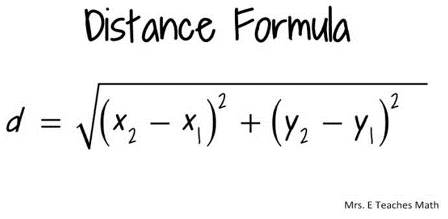# Calculating distance, area, and perimeter

Determine the distance a heron flies from its nesting colony to places it feeds. Using Google Earth, determine the GPS coordinates (in UTMs) of each place it visits, including its nesting colony. Using the distance formula, calculate straight line distances between the locations. If the bird did not fly in a straight line, use the Ruler tool in Google Earth to measure the length of the path. How far does the bird travel before it returns to the colony? How far does it travel in one day? Does it travel in a straight line or does it meander or zigzag before stopping? If it does not travel in a straight line, what could be some reasons for this? What are the sizes of the wetlands it visits – determine the area perimeter of each. Is there a preference for a certain size or shape of wetland it uses for foraging? Suggested resources:

• To measure distance:
• If you have the GPS coordinates of two locations in UTMs, you can use the following formula to calculate the distance between the two points (x1, y1) and (x2, y2):• In Google Earth, use the measuring icon on the toolbar (shown below), or go to the Tools menu and click on Ruler.